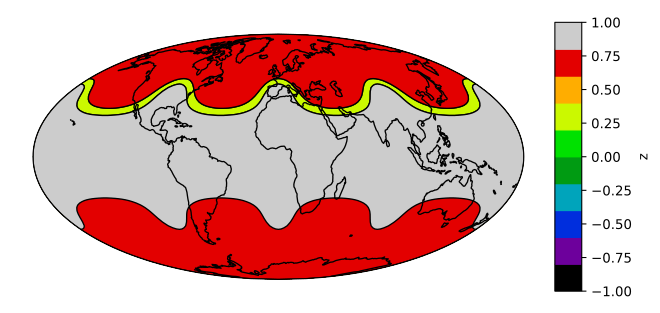# Filled Contours#

```import numpy as np
import geoviews as gv
import geoviews.feature as gf
import cartopy.crs as ccrs

gv.extension('matplotlib')

gv.output(fig='svg', size=200)
```## Define data#

```def sample_data(shape=(73, 145)):
"""Returns ``lons``, ``lats`` and ``data`` of some fake data."""
nlats, nlons = shape
ys = np.linspace(-np.pi / 2, np.pi / 2, nlats)
xs = np.linspace(0, 2 * np.pi, nlons)
lons, lats = np.meshgrid(xs, ys)
wave = 0.75 * (np.sin(2 * lats) ** 8) * np.cos(4 * lons)
mean = 0.5 * np.cos(2 * lats) * ((np.sin(2 * lats)) ** 2 + 2)

data = wave + mean

return lons, lats, data

lons, lats, data = sample_data()

# Make sure we declare the central longitude
contours = gv.FilledContours((lons, lats, data), crs=ccrs.PlateCarree(central_longitude=180))
```

## Plot#

```contours.opts(colorbar=True, levels=8, color_levels=10, cmap='nipy_spectral', projection=ccrs.Mollweide()) * gf.coastline
```This web page was generated from a Jupyter notebook and not all interactivity will work on this website. Right click to download and run locally for full Python-backed interactivity.